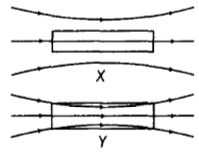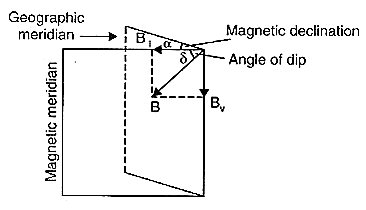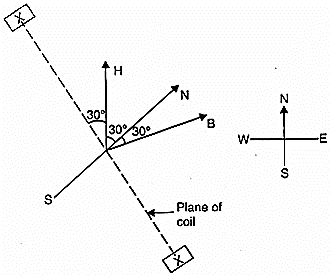# CBSE Class 12 Physics Magnetism and Matter Important Questions## myCBSEguide App

Complete Guide for CBSE Students

NCERT Solutions, NCERT Exemplars, Revison Notes, Free Videos, CBSE Papers, MCQ Tests & more.

CBSE Class 12 Physics Magnetism and Matter Important Questions. We know Physics is tough subject within the consortium of science subjects physics is an important subject. But if you want to make career in these fields like IT Consultant, Lab Technician, Laser Engineer, Optical Engineer etc. You need to have strong fundamentals in physics to crack the exam. myCBSEguide has just released Chapter Wise Question Answers for class 12 Physics. There chapter wise Extra Questions with complete solutions are available for download in myCBSEguide website and mobile app. These Questions with solution are prepared by our team of expert teachers who are teaching grade in CBSE schools for years. There are around 4-5 set of solved Physics Extra Questions from each and every chapter. The students will not miss any concept in these Chapter wise question that are specially designed to tackle Board Exam. We have taken care of every single concept given in CBSE Class 12 Physics syllabus and questions are framed as per the latest marking scheme and blue print issued by CBSE for class 12.

Class 12 Physics Extra Questions

## Chapter 5 Class 12 Physics Latest Exam Questions

Class – 12 Physics (Magnetism and Matter)

1. A long solenoid with 80 turns of wire per centimeter carries a current of 0.15 A. The wire that makes up the solenoid is wrapped around a solid core of silicon steel Km = 5200 (The wire of the solenoid is jacketed with an insulator so that none of the current flows into the core.) the magnetization inside the core is

1. 6.24 MA/m
2. 5.84 MA/m
3. 6.48 MA/m
4. 6.04 MA/m
2. Relative permeability of iron is 5500. Its magnetic susceptibility is:

1. 5500 {tex}\times{/tex} 10-7
2. 5499
3. 5501
4. 5500 {tex}\times{/tex} 107
3. A Rowland ring of mean radius 15 cm has 3500 turns of wire wound on a ferromagnetic core of relative permeability 800. What is the magnetic field B in the core for a magnetising current of 1.2A?

1. 3.48 T
2. 5.48 T
3. 4.08 T
4. 4.48 T
4. A short bar magnet of magnetic moment {tex}{\rm{5}}.{\rm{25 }} \times {\rm{ 1}}{0^{ – {\rm{2}}}}{\rm{J }}{{\rm{T}}^{ – {\rm{1}}}}{/tex} is placed with its axis perpendicular to the earth’s field direction. At what distance from the centre of the magnet, the resultant field is inclined at 45 degree with earth’s field on its axis. Magnitude of the earth’s field at the place is given to be 0.42 G. Ignore the length of the magnet in comparison to the distances involved.

1. 6.3 cm
2. 7.3 cm
3. 5.8 cm
4. 6.8 cm
5. According to Gauss’s law for magnetism

1. {tex}\oint \vec{B}.\vec{ds} = 0{/tex}
2. {tex}\int \vec{B}.\vec{ds} = 0{/tex}
3. {tex}\oint \vec{B}.\vec{ds} = \mu_0{/tex}
4. {tex}\int \vec{B}.\vec{ds} = \mu_0{/tex}
6. Where on the surface of earth is the vertical component of earth’s magnetic field zero?

7. At a place, the horizontal component of earth’s magnetic field is B and angle of dip is 60°. What is the value of the earth’s magnetic field at equator?

8. The hysteresis loop of a soft iron piece has a much smaller area than that of a carbon steel piece. If the material is to go through repeated cycles of magnetization, which piece will dissipate greater heat energy?

9. If the solenoid is free to turn about the vertical direction and a uniform horizontal magnetic field of 0.25 T is applied, what is the magnitude of torque on the solenoid when its axis makes an angle of 30° with the direction of applied field?

10. What is the susceptibility and permeability of a perfectly diamagnetic substance?

11. Distinguish between diamagnetic and ferromagnetic materials in terms of

1. susceptibility and
2. their behaviour in a non-uniform magnetic field.
12. A circular coil of 16 turns and radius 10 cm carrying a current of 0.75 A rests with its plane normal to an external field of magnitude {tex}5.0 \times {10^{ – 2}}T{/tex}. The coil is free to turn about an axis in its plane perpendicular to the field direction. When the coil is turned slightly and released, it oscillates about its stable equilibrium with a frequency of 2.0s-1. What is the moment of inertia of the coil about its axis of rotation?

1. How does angle of dip change as line goes from magnetic pole to magnetic equator of the earth?
2. A uniform magnetic field gets modified as shown in the figure below, when two specimens X and Y are placed in it. Identify whether specimens X and Y are diamagnetic, paramagnetic or ferromagnetic.13. Explain with the help of diagram the terms

1. magnetic declination and
2. angle of dip at a given place.
14. A tangent galvanometer is set with its plane making an angle of 30° with the magnetic meridian. When a current is passed through 2 turns coil of radius 0.10 m, in the anti-clockwise direction, the compass needle shows a deflection of 30°. Calculate the strength of current passed. Take H {tex} = 0.32 \times {10^{ – 4}}T{/tex}.

Class – 12 Physics (Magnetism and Matter)

1. 6.24MA/m
Explanation: {tex}M={B\over \mu_o}={\mu_oK_mNI\over \mu_o}{/tex}
{tex}=5200\times 80\times 10^2\times 0.15{/tex}
{tex}=6.24\times 10^6A/m{/tex}
1. 5499
Explanation: {tex}\chi_m=\mu_r-1=5500-1=5499{/tex}
1. 4.48 T
Explanation: {tex}B={\mu_o\mu_rNi\over2\pi r}={4\pi\times 10^{-7}\times 800\times 3500\times 1.2\over 2\pi\times 15\times 10^{-2}}{/tex} = 4.48 T
1. 6.3 cm
Explanation: The magnetic field at a distanced Rr from the centre of the magnet on its axis is given as:
{tex}{B^1} = \frac{{{\mu _0}2M}}{{4\pi {R^3}}}{/tex}
The resultant field is inclined at 45°with earth’s field.
{tex}\therefore \;{B^r} = H{/tex}
{tex}\frac{{{\mu _0}2M}}{{4\pi {{({R^r})}^3}}} = H{/tex}
{tex}{({R^r})^3} = \frac{{{\mu _0}2M}}{{4\pi \times H}}{/tex}
{tex} = \frac{{4\pi \times {{10}^{ – 7}} \times 2 \times 5.25 \times {{10}^{ – 2}}}}{{4\pi \times 0.42 \times {{10}^{ – 4}}}} = 25 \times {10^{ – 5}}{/tex}
{tex}\therefore{/tex} Rr = 0.063m = 6.3 cm
1. {tex}\oint \vec{B}.\vec{ds} = 0{/tex}
Explanation: Since magnetic monopoles do not exist, flux entering the closed surface is equal to flux leaving the surface. Hence net magnetic flux through a closed surface is zero.
1. At magnetic equator, vertical component of earth’s magnetic field will be zero. As vertical component of field is Bv = Bsinδ and at equator δ = 0o
2. Let {tex}B_E{/tex} is earth’s magnetic field
Now, {tex}B_E\cos 60 ^ { \circ } = B \Rightarrow B_E = \frac { B } { \cos 60 ^ { \circ } } = \frac { B } { 1 / 2 } = 2 B{/tex}
3. Carbon steel piece, because heat lost per cycle is proportional to the area of hysteresis loop.
4. Given, N = 800, I = 3 A, A = {tex}2.5 \times {10^{ – 4}}{m^2}{/tex}
B = 0.25 T, {tex}\theta = 30^\circ {/tex}
Magnetic moment, M = N I A
{tex} = 800 \times 3.0 \times 2.5 \times {10^{ – 4}}{/tex}
= 0.5 JT-1
Torque, {tex}\tau = MB\;\sin \theta = 0.6 \times 0.25 \times \sin 30^\circ {/tex}
{tex} = 0.150 \times 0.5 = 7.5 \times {10^{ – 2}}J{/tex}.
5. For a perfectly diamagnetic substance.
{tex}B = {\mu _0}\left( {H + I} \right) = 0{/tex}
I = -H
Therefore {tex}{\chi _m} = \frac{{ – H}}{H} = – 1{/tex}
Also {tex}{\mu _r} = 1 + {\chi _m} = 1 – 1 = 0{/tex}
{tex}\therefore \mu = {\mu _0}{\mu _r}{/tex}= zero

1. Susceptibility for diamagnetic material
It is independent of magnetic field and temperature (except for bismuth at low temperature).
Susceptibility for ferromagnetic material
The susceptibility of ferromagnetic materials decreases steadily with increase in temperature. At the Curie temperature, the ferromagnetic materials become paramagnetic. The value of magnetic succeptibility is positive and much more greater than 1 for ferromagnetic materials.
2. Behaviour in non-uniform magnetic field
Diamagnets are feebly repelled, whereas ferromagnets are strongly attracted by non-uniform field, i.e. diamagnets move in the direction of decreasing field, whereas ferromagnet feels force in the direction of increasing field intensity.
6. Here, N = 16, r = 10 cm = 0.1 m
I = 0.75 A, {tex}B = 5.0 \times {10^{ – 2}}T{/tex}
v = 2.0 s-1
M = NIA {tex} = NI\pi {r^2}{/tex}
{tex} = 16 \times 0.75 \times \frac{{22}}{7}{(0.1)^2}{/tex}
{tex} = 0.377\;J{T^{ – 1}}{/tex}
Using formula
{tex}v = \frac{1}{{2\pi }}\sqrt {\frac{{MB}}{I}} {/tex}
{tex}\therefore {v^2} = \frac{{MB}}{{4{\pi ^2}I}}{/tex}
Or {tex}I = \frac{{MB}}{{4{\pi ^2}{v^2}}}{/tex}
Putting values, {tex}I = \frac{{0.377 \times 5.0 \times {{10}^{ – 2}}}}{{4 \times {{\left( {\frac{{22}}{7}} \right)}^2} \times {2^2}}} = 1.2 \times {10^{ – 4}}kg\;{m^2}{/tex}

1. The angle of dip decreases from 90°to 0° as we move from pole to the equator of earth.
2. For paramagnetic materials, magnetic lines of force are feebly attracted by the materials and for that reason only a few magnetic field lines enter in it. So, specimen X is paramagnetic. For ferromagnetic materials, all magnetic lines of force prefer to go through it. So, specimen Y is ferromagnetic.
1. Magnetic declination at a place may be defined as the angle between its magnetic meridian and the earth geographic meridian at the place.2. Angle of dip at a place is defined as the angle between the direction of intensity of earth’s magnetic field (BE) and the horizontal direction in magnetic meridian at that place.
7. Before passing current, compass needle was along H. On passing current, the needle shows a deflection of 30° (with H) under the combined effect of H and B, magnetic field due to current (in a direction perpendicular to the plane of the coil).
As compass needle is inclined equally to H and B, figure, therefore.
B = H
{tex}\frac{{{\mu _0}NI}}{{2r}} = H{/tex}
{tex}I = \frac{{2rH}}{{{\mu _0}N}} = \frac{{2 \times 0.1 \times 0.32 \times {{10}^{ – 4}}}}{{4\pi \times {{10}^{ – 7}} \times 2}}{/tex}
or I = 2.54 A## Chapter Wise Extra Questions of Class 12 Physics Part I & Part II## Test Generator

Create Papers with your Name & Logo